Search

# Complex Numbers and Fluid Flow - are they related? (Part I)

This would be a short article if the answer was no, but with this we now ask. "But how are they related?"

In this part, I'll discuss the idea of a complex function, and a rule that must be followed for one to have a derivative.Note: This article does require some prior understanding on complex numbers and high school derivatives. But other than that, I'll put things in the simplest way I can. Even if you don't know, hopefully this will allow you to paint a mental image of them.

Many people get the idea of a function. Many people also know what a complex number is. But not as many have stopped to think of the idea of a complex function. In short it is simply a regular function that takes in a complex number and spits out another. In fact, likely every function you have dealt with can be used as a complex function. Instead of considering a function with 1 input and 1 output (y as an output and x as an input)We consider a function with 2 inputs and 2 outputs (u and v as outputs and x and y as inputs). For convenience of understanding the "i", one way to think about it is that it makes whatever its multiplied to vertical. v is a vertical part, and so is y (read on and it should make sense)Note that both u and v depend on both x and y. This can be further simplified asWhere w=u+iv and z=x+iy. This is exactly the same format as y=f(x) but simply that w and z are complex numbers, whereas x and y are purely real. To plot this, we would need 4 dimensions (2 for x&y, and 2 for u&v). But we can't do that in our 3-D world. But people are creative. One way is to use something called a vector field like below:For each point (x and y value), the value of u and v is represented in a vector (an arrow). u takes care of the horizontal component and v takes care of the vertical component(see diagram below). Generally these vector fields get crowded so they are scaled down so it doesn't seem too overwhelming.(forget the 1's on the bottom for x and y, they are a technical issue)

So u and v change the vector, and they depend on what x and y are.

Now let's discuss about what it means to take a derivative of a complex function. The general idea of a derivative is that if I move my input by a tiny amount, dx, how big is my corresponding change to my output? And in high school you may have been taught that it as the slope of a curve. But that isn't the only way to represent it...

Consider the number line belowThe vector represents the y=x², where the length of the vector is the square of the point it starts from. For example if I were at the point x=2, then the length of the vector is 2²=4. You can then imagine all vectors for every possible number. Obviously this would overcrowd the number line, so these would have to be scaled down and spaced out. You can then think of the derivative as asking

"If I change my position (position of point A) by a tiny amount, dx, by how much does the vector change?" This doesn't seem like a special nor practical way of viewing derivatives, but what it does let us do is give us a platform for seeing derivatives for complex numbers.

Now when we translate this into a derivative of a complex function, it asks the same question: "If I change my input by a tiny amount how does it change my output?"Here we are changing the value of our input (the point where we "measure" the vector) and seeing the resulting change in the vector. The more horizontal, the bigger the value of u, and the more vertical, the bigger the value of v. The aim is to imagine if we make really small changes to the input, so instead of changing our input by 1 in the diagram (in either horizontal or vertical directions, or any direction for that matter), we imagine changing our input by a tiny amount, dz.

The derivative of a complex function asks for the change in a vector when you slightly shift the position of the point you are measuring. Does the vector turn? Does it enlarge or contract? And by how much?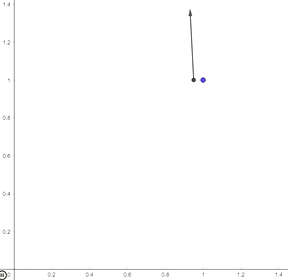Another way of viewing this is by asking the "speed" of the vector as it is close to a particular point. Consider the two moving vectors above. One moves horizontally near the point (1,1) and the other moves past the same point but vertically. Notice how they grow at the same speed? and that they rotate at the same speed? It doesn't care which direction you approach the point, the speeds still stay the same. If a complex function can achieve this, we call it differentiable.

Now you might be thinking "Isn't every function differentiable?". Most, if not all functions you deal with in high school are differentiable, and it may lead one to think that practically any continuous function is differentiable. Here is an example of a function that isn't differentiable: (u+iv)=x+ix. It looks like this, and observe the vectors (arrows) moving across the point 1,1 below.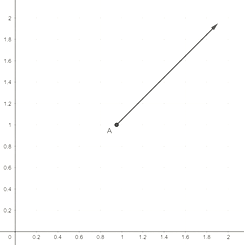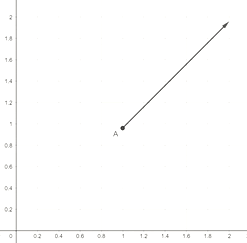Notice how the arrow grows slightly when the point is moved horizontally (also shown on the vector field), but doesn't change at all when moved vertically? The speed when crossing the same point is different in different directions, so we say it isn't differentiable.

Now for the fun part, to transfer this from intuition into the language of maths!

Firstly, we said that complex functions can be broken into real and imaginary components.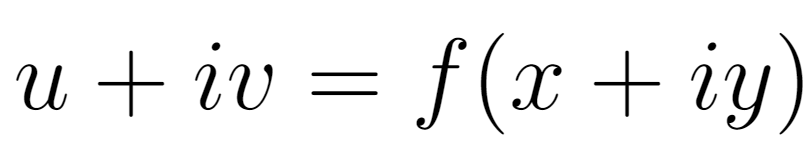Both u and v depend on both x and y, so I can also rewrite it like thisThis doesn't change anything really, but it just reminds us that both u and v depend on x and y. Taking derivatives with respect to z, we getRemember that this is simply asking the "speed" of the vector changing as we hover near any particular point. Now I can split the right side like soSimply put, all we have done is split the total speed into horizontal speed (the du/dz) and the vertical speed (the dv/dz). Again the i simply tells us that we are working in the vertical direction.

Now this is where things get interesting. du/dz asks if we hover over a particular point, going in any direction how does the horizontal speed of the vector change? Now we can consider any particular direction and we know that this speed must stay the same if this derivative is to have one value. Lets consider the horizontal direction. What I mean to ask is

"If I hover over a particular point horizontally, what is the horizontal speed of the vector?"We (at point A) are hovering horizontally over our particular point P, and the function creates our vector which moves diagonally. What I am asking is the speed of F (in red), which moves horizontally in line with B.

Going back to calculus, we take limits when we consider our derivativeNow that lim business on the right side simply asks what does the speed become as we hover over point P but over a smaller and smaller interval. Imagine it with the gif and images below as a guide:Now this is represented as a derivative with the curly d's like soThis derivative is simply the same as the limit but contracted.

Now we can also consider the point hovering vertically over the particular point, and then measuring the change (speed) in u (the horizontal speed of the vector), and it looks like thisThis uses the property that 1/i=-i, and the derivative works the same way as before, but only that the hovering we are doing is purely vertical.

Likewise we can now apply similar limits for measuring dv/dz like so:Now we can get two different ways to represent df/dz (the overall speed of a vector as we hover over a particular point, vertically or horizontally)Now we have two equations that are equal to the same thing, df/dz. So we can equate them.A special thing about equations with complex numbers is that all the real parts (parts that aren't multiplied with i) are equal to each other, and all the imaginary parts are equal to each other (parts that are multiplied with i). So we finally get this pair of equations (I cancelled the i's and shifted the - sign on the bottom equation):These pair of equations is the raw product! They are called the Cauchy Riemann equations, and basically tell us if a complex function has a derivative or not. If a complex function has a derivative, they satisfy these equations.

As a quick example, lets test the function f(z)=z² . If I expand it so that z=x+iy, I getSo by equating real and imaginary parts we get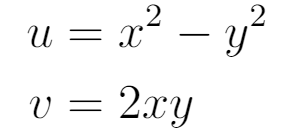Now for all these derivatives with the curly d's (known as partial derivatives). The way these work is that it treats all other letters as constant, and you differentiate only with respect to the letter on the bottom, like soBy substitution you can easily see that these satisfy the Cauchy Riemann equations above. This concludes the complex numbers section of the series. Next part we talk about a special type of fluid flow, and the equations that govern it. See you next time!